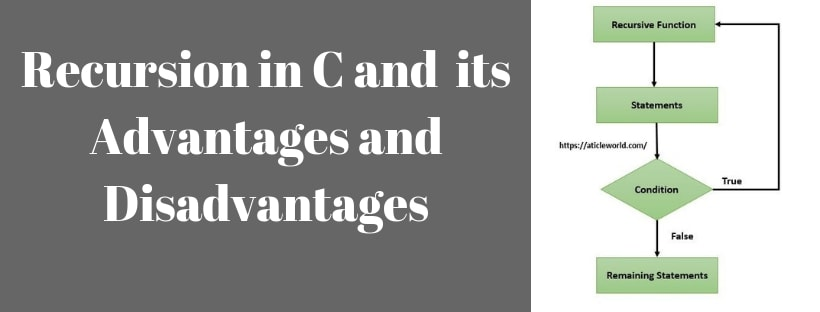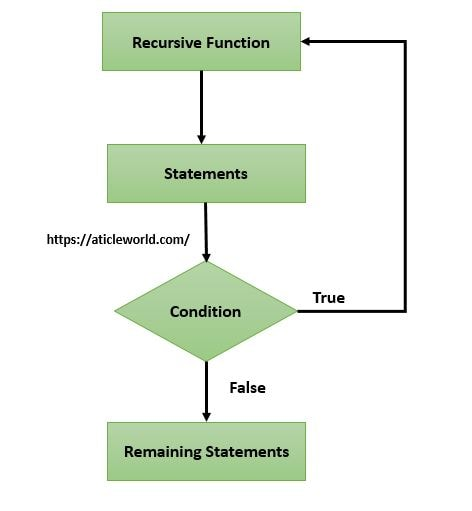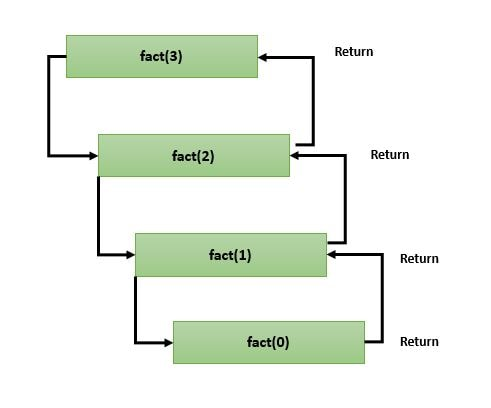# Recursion in CRecursion is a process in which function call itself and the function that calls itself directly or indirectly called recursive function. A recursive function calls itself so there can be several numbers of the recursive call, so the recursive function should have the termination condition to break the recursion. If there is a not termination condition in a recursive function, a stack overflow will occur and your program will crash.

Recursive functions are useful to solve many mathematical problems, like generating Fibonacci series, calculating factorial of a number and convenient for recursively defined data structures like trees.

Let’s see an example,

In the above code, test() is a recursive function that calls itself. You can see, if you will not put termination condition in test(), stack-overflow will occur. So we have to put a condition in test() to avoid an infinite loop (until not stack overflow occurs).

See the below code,### Direct vs. Indirect Recursion

A direct recursive function is a function which is called itself.

See the below code,

A function is called indirect recursive if it calls another function and the called function call the calling function.

For example, Test() is called the called indirect recursive function if it calls another function to say Test1() and Test1() calls Test() directly or indirectly.

##### If you want to learn more about the c language, here 10 Free days C video course for you.### How does recursion work?

If you are going to create a recursive function then you should remember that each invocation of the recursive function creates a fresh set of all automatic variables.

Let see a code to understand the working of the recursive function.

In below code, I am creating a recursive function to calculate the factorial of a number. Basically, factorial is the product of the all positive number from 1 to n (n is the number). In simple word you can say that factorial of n would be 1*2*3*…..*n.

Factorial of positive number would be:

Note: !0 and !1 will be 1

#### Code to calculate factorial of a number using recursion in C

Before writing the code I want to show here a flow diagram which to describe the flow of the code.### Working of the above code,

Here n = 3

fact(3) = 3 * fact(2)
fact(2) = 2* fact(1)
fact(1) = 1 *fact(0);

When n=0, condition becomes true and recursion stops and control returns to factorial(1). Now reverse process occurs and function will return a value to the previous function calls.So final result will be:

fact(3) = 3*2*1 = 6

A recursive function has a lot of advantage and disadvantage. here I am mentioning few advantage and disadvantage of the recursive function.

1. Using the recursion you can make your code simpler and you can solve problems in an easy way while its iterative solution is very big and complex.

2. Recursive functions are useful to solve many mathematical problems, like generating Fibonacci series, calculating factorial of a number.

3. Recursive functions convenient for recursively defined data structures like trees.

1. Recursion makes your code slow because each time needs to create a stack frame for the function call.

2. Recursion takes a lot of stack memory because it requires the stack space in every invocation.

3. A recursive function should have a base condition to break the recursive calling of the function either it will cause of the stack overflow.

4. If recursion is too deep, then there is a danger of running out of space on the stack and it can cause of the program crashes.

### Recursion vs Iteration

1. Due to the overhead of maintaining the stack usually, recursion is slower as compared to the iteration.

2. Usually, recursion uses more memory as compared to the iteration.

3. Sometimes it is much simpler to write the algorithm using recursion as compared to the iteration.

If you have any doubt regarding the recursive function, please write the comment in comment box. I will resolve your problem as soon as possible.